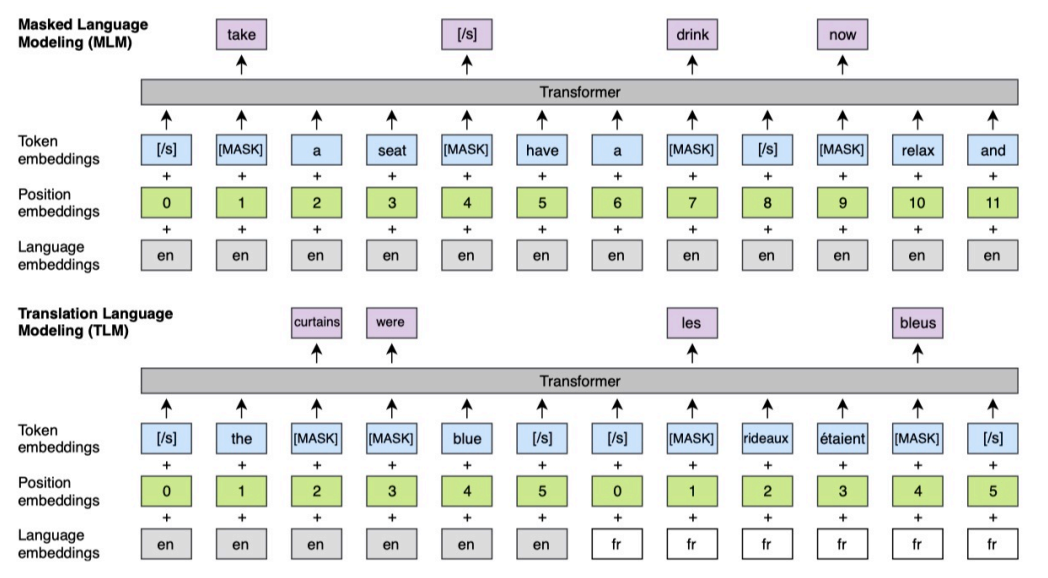# 基于统计的方法

https://zhuanlan.zhihu.com/p/45730674
https://papers.nips.cc/paper/5477-neural-word-embedding-as-implicit-matrix-factorization.pdf
https://cloud.tencent.com/developer/article/1436217

• 共现次数：$p(y | x)=\frac{p(x, y)}{p(x)} \approx \frac{c(x, y)}{c(x)} \propto c(x, y)$
• 互信息：\begin{aligned} \operatorname{PMl}(x, y) &=\log \frac{p(x, y)}{p(x) p(y)} \\ & \approx \log \frac{c(x, y)}{c(x) c(y)} \propto \log c(x, y)-\log c(y) \end{aligned}

• Controlled Predictive Effects（CPE）：\begin{aligned} \operatorname{CPE}(y | x) &=p(y | x)-\sum_{x^{\prime} \in \mathcal{X}} p\left(y | x^{\prime}\right) p\left(x^{\prime} | x\right) \\ &=\sum_{x^{\prime} \in \mathcal{X}} \operatorname{CPE}_{y | x}\left(x^{\prime}\right) p\left(x^{\prime} | x\right) \end{aligned}

## 互信息

PMI是NLP中的一个重要指标，在本文中它可以用来阻止一些stop word有较高的得分。互信息是信息论里一种有用的信息度量，它可以看成是一个随机变量中包含的关于另一个随机变量的信息量，或者说是一个随机变量由于已知另一个随机变量而减少的不肯定性。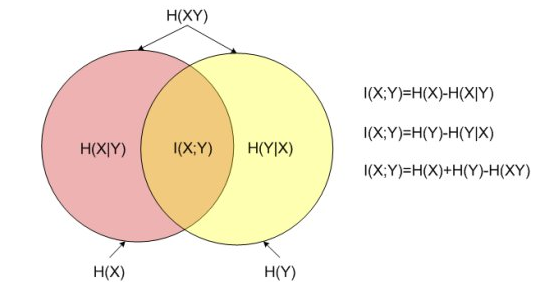• word: $w \in V_{W}$
• context: $c \in V_{C}$
• $w_i$的context为其窗口长度为L的附近的词：$w_{i-L}, \ldots, w_{i-1}, w_{i+1}, \ldots, w_{i+L}$
• word vocabulary: $V_w$
• context vocabulary: $V_c$
• word和context的pair集合：$D$
• (w, c)在D中出现的次数：$c(w,c)$
• w在D中出现的次数：$c(w)=\sum_{c^{\prime} \in V_{C}} c\left(w, c^{\prime}\right)$
• c在D中出现的次数：$c(c)=\sum_{w^{\prime} \in V_{W}} c\left(w^{\prime}, c\right)$
• 每个词w可以表示成向量：$\vec{w} \in \mathbb{R}^{d}$，有时用$\left|V_{W}\right| \times d$的矩阵W表示，其中$W_i$表示第$i$个word的表示
• 每个context c可以表示成向量：$\vec{c} \in \mathbb{R}^{d}$，有时用$\left|V_{C}\right| \times d$ 的矩阵C表示，这里的d表示embedding的维度，其中$C_i$表示第$i$个context的表示
• (w, c)属于D的概率：$P(D=1 | w, c)$

【题外话】：早期的word2vec也有基于词-词共现矩阵的方法，使用LSA将从corpus中统计的word-word共现矩阵进行分解。共现矩阵里的值有时用PMI替代：$P M I\left(w_{i}, w_{j}\right)=\log \frac{p\left(w_{i}, w_{j}\right)}{p\left(w_{i}\right) p\left(w_{j}\right)}=\log \frac{c\left(w_{i}, w_{j}\right) \cdot|\mathcal{C}|}{c\left(w_{i}\right) \cdot c\left(w_{j}\right)}$。使用SVD对矩阵分解得到：$\mathbf{P}=\mathbf{U} \Psi \mathbf{V}^{\top}$，那么当我们想得到K维的，就可以计算：$\mathbf{X}=\mathbf{U}_{k} \mathbf{\Psi}_{k}$。

## CPE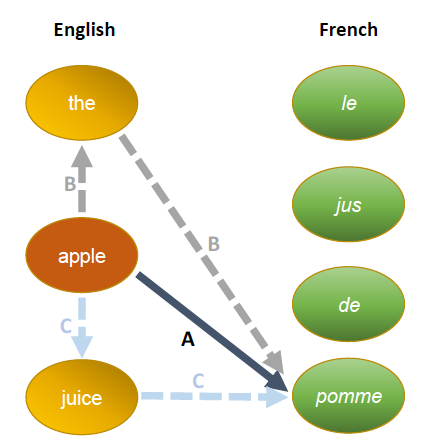## 源码解读

### get_trans_co

get_trans_co用于获取x->y共现最多的pair，传入参数x2ys已计算好x->y的共现次数。

### get_trans_pmi

get_trans_pmi用于对x的所有translation y进行pmi排序。

### rerank

rerank用于对x的所有translation y进行cpe排序。

### rerank_mp

rerank_mp使用多进程方式进行CPE重排序。

# 基于词向量的方法

（1）A Survey Of Cross-lingual Word Embedding Models
https://arxiv.org/abs/1706.04902
http://ir.hit.edu.cn/~xiachongfeng/slides/x-lingual-v1.0.pdf
https://zhuanlan.zhihu.com/p/69366459
（2）Word Translation Without Parallel Data
https://arxiv.org/abs/1710.04087
（3）Massively Multilingual Sentence Embeddings for Zero-Shot Cross-Lingual Transfer and Beyond
https://arxiv.org/abs/1812.10464
https://github.com/yannvgn/laserembeddings
（4）Loss in Translation: Learning Bilingual Word Mapping with a Retrieval Criterion
https://arxiv.org/pdf/1804.07745.pdf
https://fasttext.cc/docs/en/aligned-vectors.html

## 跨语言词向量综述

A Survey Of Cross-lingual Word Embedding Models这篇论文中对跨语言的词向量的相关研究进行了总结，并且发现很多模型其实本质上是一个模型，只是使用了不同的目标函数和数据。为了方便理解，我们先将后续用到的符号进行说明：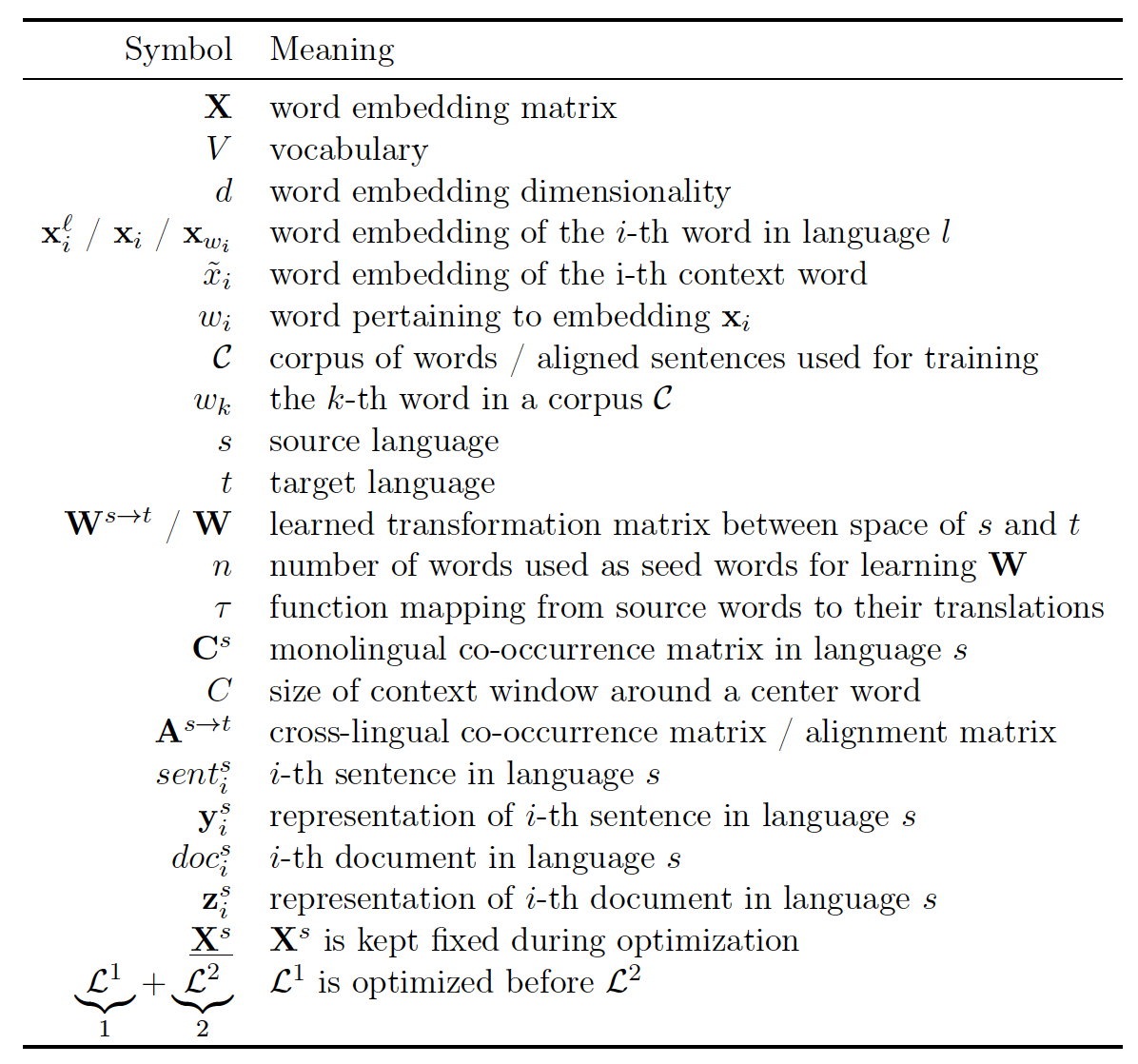### Typology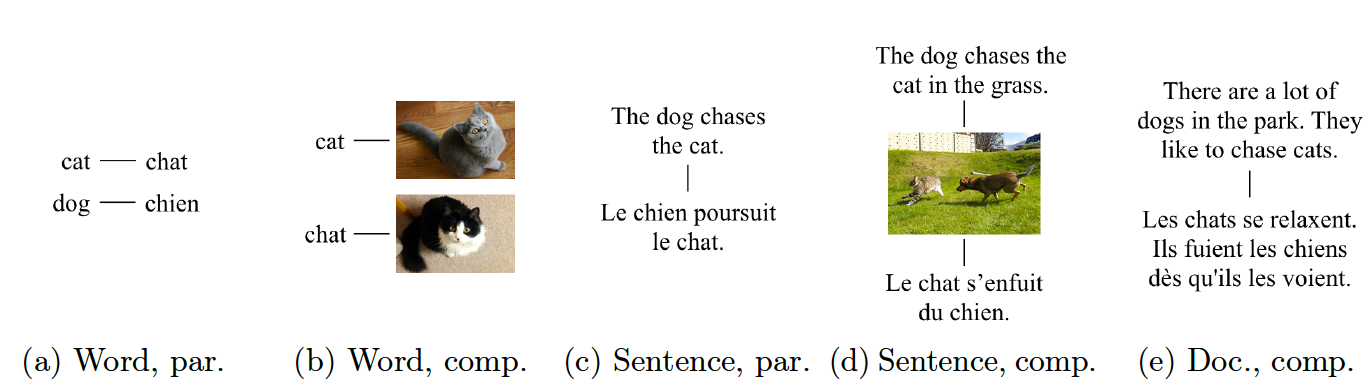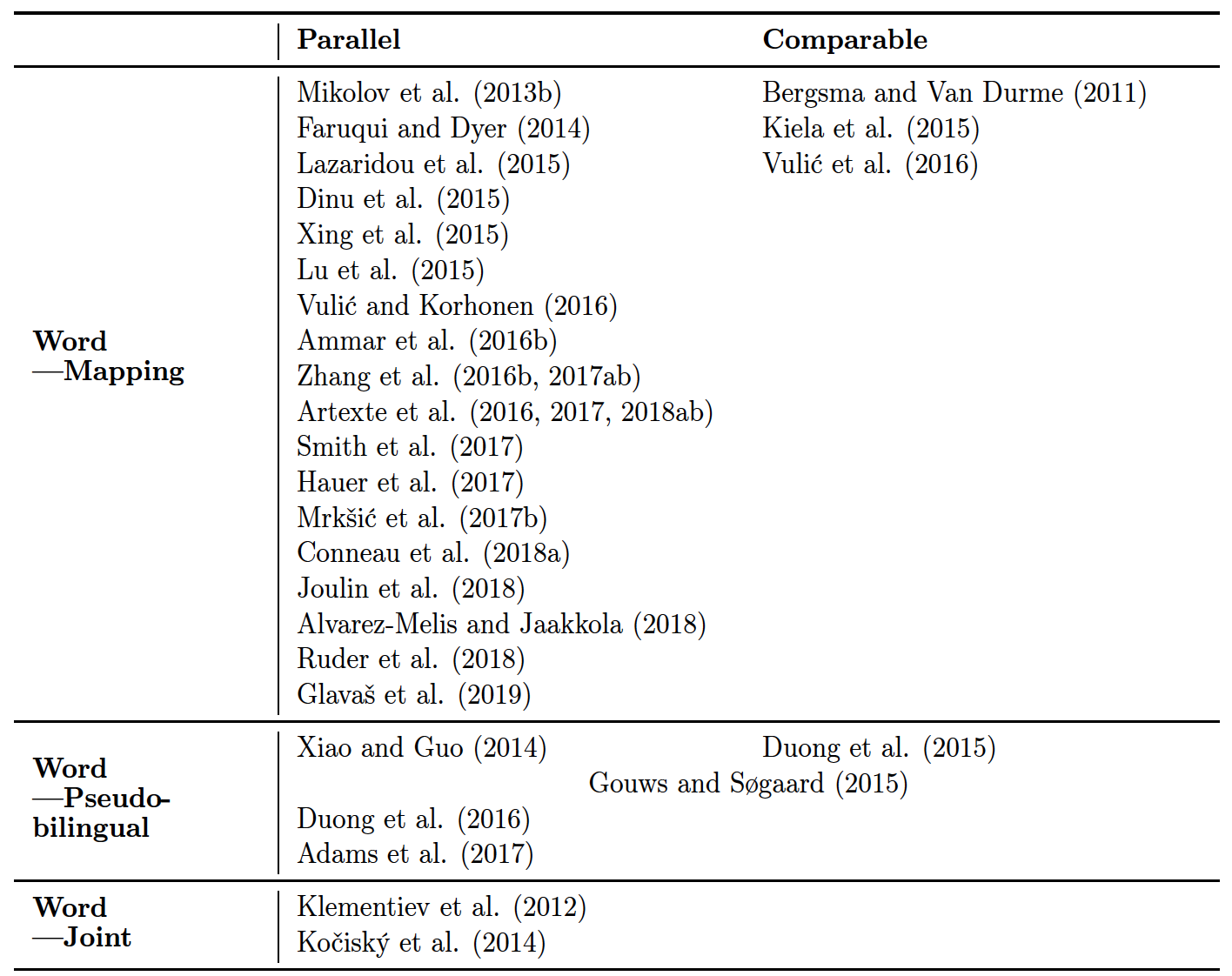### Word-Level Alignment Models

• Mapping-based：通过学习平行语料或者平行词典的映射关系，现训练一个源语言的embedding，再用学习到的映射矩阵映射到目标语言词向量空间中
• Pseudo-multi-lingual corpora-based：用跨语言的伪语料来捕捉不同语言单词间的相互作用，这个伪语料是人工构造的。例如根据翻译，可以定义英语 house 和法语 maison 是等价 的，根据词性标注，可以定义英语 car 和法语 maison 都是名词是等价的。因此这里的对齐方式不一 定是翻译，可以根据具体的任务来定义，然后利用这种对齐关系来构造双语伪语料。首先将源语言 和目标语言数据混合打乱。对于统一语料库中一句话的每一个词语，如果存在于对齐关系中，以一 定概率来替换为另一种语言的词语。通过该方法可以构建得到真实的双语语料库。例如根据翻译关 系，原始句子 build the house 经过构建可以得到 build the maison，就是将 house 替换为了 maison。 利用构建好的全部语料来使用 CBOW 算法学习词向量，由于替换以后的词语有相似的上下文，因 此会得到相似的表示。对于那些没有对齐关系的词语，例如“我吃苹果”和“I eat apple”，吃和 eat 没有对齐关系，但如果我和 I、苹果和 apple 有对齐关系，根据构造出来的语料“I 吃 apple”也可 以完成吃和 eat 的隐式对齐。这种方法对齐词语有相似表示。
• Joint-method：利用平行语料来最小化monolingual losses + cross-lingual regularization term

• Language grounding models：把图片作为anchor，通过图片特征来获得language similarity的特征
• Comparable feature models：利用POS信息建立两个language的桥梁

• mapping method：负责将monolingual embedding spaces转换到cross-lingual embedding space。Mapping method有以下几种方法：
• Regression methods
• 学习source到target的转移矩阵，并最大化source embedding和target embedding的相似度：$\Omega_{\mathrm{MSE}}=\sum_{i=1}^{n}\left|\mathbf{W} \mathbf{x}_{i}^{s}-\mathbf{x}_{i}^{t}\right|^{2}$，其中$x^s_i$是source embedding，经过W转换后，希望最小化它和其真正的翻译$x^t_i$的embedding之间的距离。论文认为这个目标函数也可以写成这种形式：$J=\underbrace{\mathcal{L}_{\mathrm{SGNS}}\left(\mathbf{X}^{s}\right)+\mathcal{L}_{\mathrm{SGNS}}\left(\mathbf{X}^{t}\right)}_{1}+\underbrace{\Omega_{\mathrm{MSE}}\left(\underline{\mathbf{X}}^{s}, \underline{\mathbf{X}}^{t}, \mathbf{W}\right)}_{2}$。Regression method的想法来源于一个观察，就是source word之间的相关性，和他们的所对应的target word之间的相关性相似，如下图：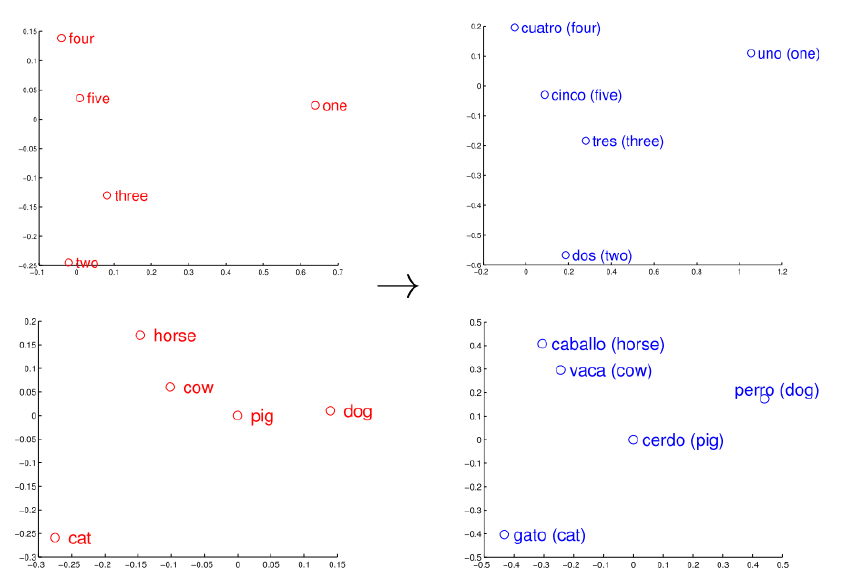• Orthogonal methods
• 同Regression method，但要求W是正交的，即$\mathbf{W}^{\top} \mathbf{W}=\mathbf{I}$
• Canonical methods
• 将source和target共同映射到另一个空间，并最大化两个embedding的相似度。我们定义映射后的两个单词的相关性为：$\rho\left(\mathbf{W}^{s \rightarrow} \mathbf{x}_{i}^{s}, \mathbf{W}^{t \rightarrow} \mathbf{x}_{i}^{t}\right)=\frac{\operatorname{cov}\left(\mathbf{W}^{s \rightarrow} \mathbf{x}_{i}^{s}, \mathbf{W}^{t \rightarrow} \mathbf{x}_{i}^{t}\right)}{\sqrt{\operatorname{var}\left(\mathbf{W}^{s \rightarrow \mathbf{x}_{i}^{s}}\right) \operatorname{var}\left(\mathbf{W}^{t \rightarrow \mathbf{x}_{i}^{t}}\right)}}$，则canonical method的目标是最大化所有的相关性：$\Omega_{\mathrm{CCA}}=-\sum_{i=1}^{n} \rho\left(\mathbf{W}^{s \rightarrow} \mathbf{x}_{i}^{s}, \mathbf{W}^{t \rightarrow} \mathbf{x}_{i}^{t}\right)$。论文认为这个目标函数等价于：$J=\underbrace{\mathcal{L}_{\mathrm{LSA}}\left(\mathbf{X}^{s}\right)+\mathcal{L}_{\mathrm{LSA}}\left(\mathbf{X}^{t}\right)}_{1}+\underbrace{\Omega_{\mathrm{CCA}}\left(\underline{\mathbf{X}}^{s}, \underline{\mathbf{X}}^{t}, \mathbf{W}^{s \rightarrow}, \mathbf{W}^{t \rightarrow}\right)}_{2}$
• Margin methods
• 该方法将Loss函数改成了margin-based rank loss来减轻hubness的问题：$\Omega_{\mathrm{MML}}=\sum_{i=1}^{n} \sum_{j \neq i}^{k} \max \left\{0, \gamma-\cos \left(\mathbf{W} \mathbf{x}_{i}^{s}, \mathbf{x}_{i}^{t}\right)+\cos \left(\mathbf{W} \mathbf{x}_{i}^{s}, \mathbf{x}_{j}^{t}\right)\right\}$，并认为此时目标函数就是：$J=\underbrace{\mathcal{L}_{\mathrm{CBOW}}\left(\mathbf{X}^{s}\right)+\mathcal{L}_{\mathrm{CBOW}}\left(\mathbf{X}^{t}\right)}_{1}+\underbrace{\Omega_{\mathrm{MML}-\mathrm{I}}\left(\underline{\mathbf{X}}^{s}, \mathbf{X}^{t}, \mathbf{W}\right)}_{2}$
• seed lexicon：用于学习embedding的种子字典
• refinement：用于修正学习到的mapping
• retrieval：用于搜索最近邻

### Sentence-Level Alignment Methods

• Word-alignment based matrix factorization approaches
• 基于Word-alignment的方法可以先用FastAlign找到词对齐关系。如果一个source word在target空间中只有一个翻译，那么这个target翻译的embedding应该是确定的一个，但如果它在target空间中有多个翻译，那么应该认为target的embedding应该是这些翻译的一个加权平均。这就是word-alignment方法的基本思路，其目标函数为：$\Omega_{s \rightarrow t}=\left|\mathbf{X}^{t}-\mathbf{A}^{s \rightarrow t} \mathbf{X}^{s}\right|^{2}$，也可以写成：$J=\underbrace{\mathcal{L}_{\mathrm{MML}}\left(\mathbf{X}^{t}\right)}_{1}+\underbrace{\Omega_{s \rightarrow t}\left(\underline{\mathbf{X}}^{t}, \underline{\mathbf{A}}^{s \rightarrow t}, \mathbf{X}^{s}\right)}_{2}$。之所以叫factorization approach是因为有的方法将$A^{s->t}$看成是共现矩阵并用Glove的目标函数进行分解
• Compositional sentence models
• 这种方法将平行句子表示的距离最小化，即$E_{\text {dist}}\left(\operatorname{sen} t^{s}, \operatorname{sen} t^{t}\right)=\left|\mathbf{y}^{s}-\mathbf{y}^{t}\right|^{2}$，其中句子的表示使用单词表示的和，使用hinge loss作为目标函数：$\mathcal{L}=\sum_{\left(s e n t^{s}, s e n t^{t}\right) \in \mathcal{C}} \sum_{i=1}^{k} \max \left(0,1+E_{\text {dist}}\left(\operatorname{sent}^{s}, \operatorname{sent}^{t}\right)-E_{\text {dist}}\left(\operatorname{sent}^{s}, s_{i}^{t}\right)\right)$，或者$J=\mathcal{L}\left(\mathbf{X}^{s}, \mathbf{X}^{t}\right)+\Omega\left(\mathbf{X}^{s}\right)+\Omega\left(\mathbf{X}^{t}\right)$
• Bilingual autoencoder models
• 从这里开始就开始无监督的工作了，Barone开始使用对抗自动编码器将源语言词嵌入转换到目标语言中，然后训练自动编码器以重建源嵌入，同时训练鉴别器以将投射的源嵌入与实际目标嵌入区分开，如下图：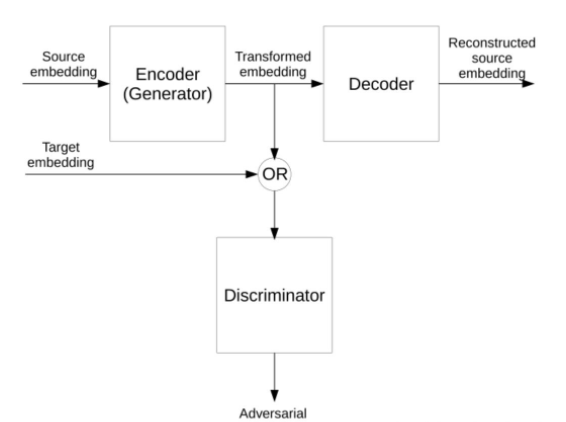• Bilingual skip-gram models

[Lample and Conneau, 2019] 提出了基于多种语言预训练的模型 XLMs，首先从单语语料库中 采样一些句子，对于资源稀少的语言可以增加数量，对于资源丰富的语言可以减少数量，将所有语 言使用统一 BPE 进行表示。使用三种语言模型目标来完成学习。前两个是基于单语语料库的，最 后一个是基于双语对齐数据的。第一种是 Causal Language Modeling (CLM)，根据之前的词语预 测下一个词语。第二个是 Masked Language Modeling (MLM)，和 BERT 类似，但是使用一个词 语流，而非句子对。第三种是 Translation Language Modeling (TLM)，可以随机 mask 掉其中一些 两种语言中的一些词语，然后进行预测。其模型如下：